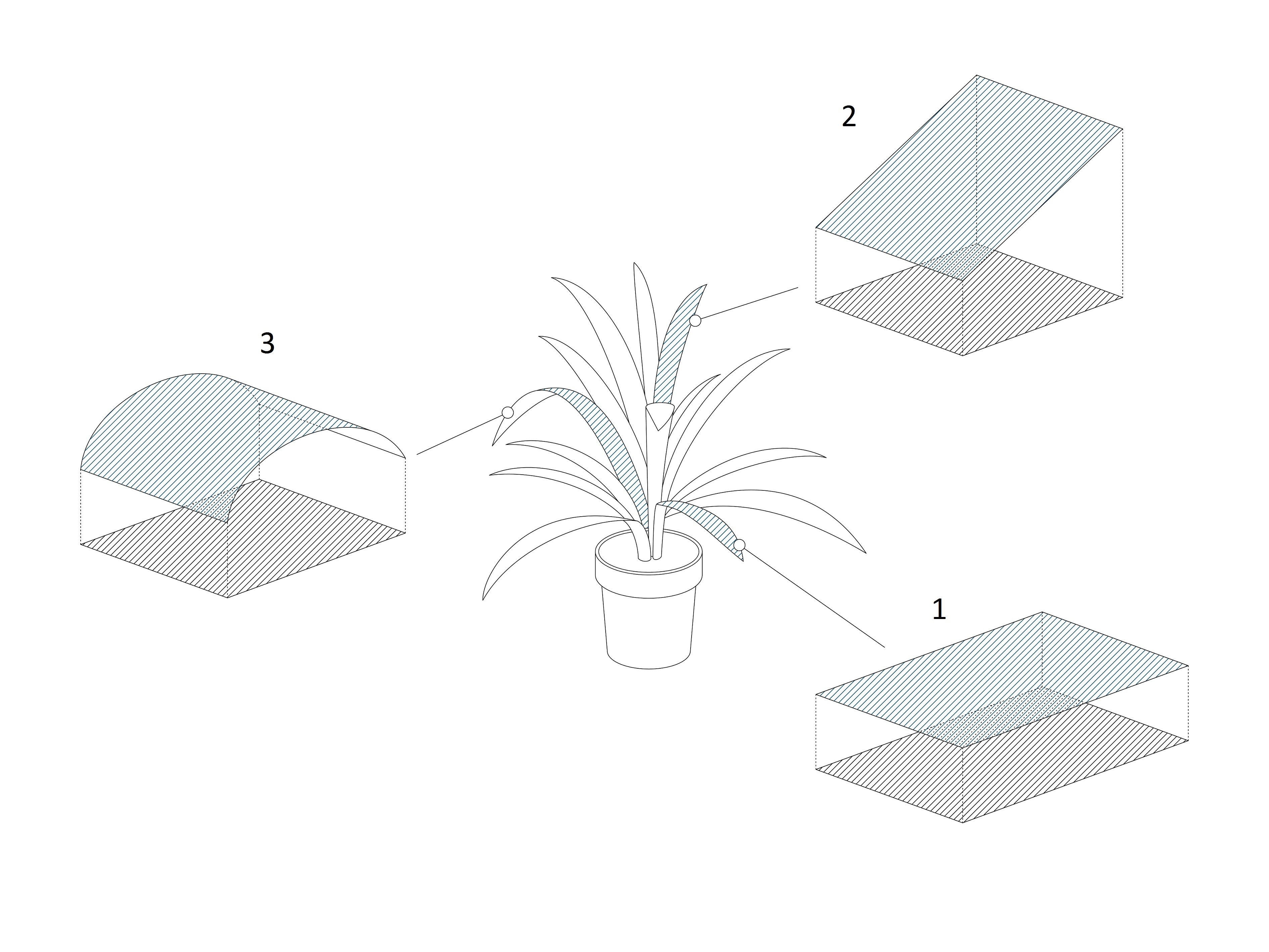Value Range: 1 – ∞ (or 0 if total leaf area is 0)
Unit: cm²/cm²

Calculated as: (Total Leaf Area)/( Proj.  Leaf Area)

Leaf inclination shall express how erected the leaves of a plant are in average. Calculated as a total leaf area divided by the sum of projections of each elementary triangle on X-Y plane. For a horizontal plane the leaf inclination would be 1. The more erected or bend a leaf is the bigger will be the total leaf area (without changing the projected leaf area) and therefore the leaf inclination will increase.The graphic above shows different examples for differently inclined leaves. Leaf number 1 has no inclination and the total leaf area is equal to the projected leaf area. Therefore, its leaf inclination is one. Leaf number 2 shows a strongly inclined leaf with a total leaf area that is 1.5 times bigger than the projected leaf area. Therefore, its leaf inclination is around 1.5. The last example number 3 shows a bent leaf. Also here the total leaf area is bigger than the projected leaf area and therefore leaf inclination is bigger than 1.

The overall leaf inclination for each plant is calculated by taking the average of all leaf inclinations for each single domain.